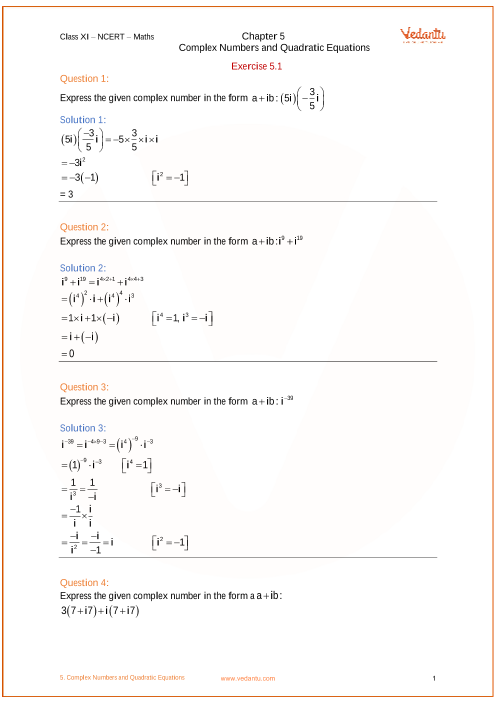# NCERT Solutions for Class 11 Maths Chapter 5 Complex Numbers and Quadratic Equations (Ex 5.2) Exercise 5.2

## NCERT Solutions for Class 11 Maths Chapter 5 Complex Numbers and Quadratic Equations (Ex 5.2) Exercise 5.2Free PDF download of NCERT Solutions for Class 11 Maths Chapter 5 Exercise 5.2 (Ex 5.2) and all chapter exercises at one place prepared by expert teacher as per NCERT (CBSE) books guidelines. Class 11 Maths Chapter 5 Complex Numbers and Quadratic Equations Exercise 5.2 Questions with Solutions to help you to revise complete Syllabus and Score More marks. Register and get all exercise solutions in your emails.

Do you need help with your Homework? Are you preparing for Exams?
Study without Internet (Offline)## NCERT Solutions for Class 11 Maths Chapter 5 Complex Numbers and Quadratic Equations Exercise 5.2

Opting for the NCERT solutions for Ex 5.2 Class 11 Maths is considered as the best option for the CBSE students when it comes to exam preparation. This chapter consists of many exercises. Out of which we have provided the Exercise 5.2 Class 11 Maths NCERT solutions on this page in PDF format. You can download this solution as per your convenience or you can study it directly from our website/ app online.

Vedantu in-house subject matter experts have solved the problems/ questions from the exercise with the utmost care and by following all the guidelines by CBSE. Class 11 students who are thorough with all the concepts from the Subject Complex Numbers and Quadratic Equations textbook and quite well-versed with all the problems from the exercises given in it, then any student can easily score the highest possible marks in the final exam. With the help of this Class 11 Maths Chapter 5 Exercise 5.2 solutions, students can easily understand the pattern of questions that can be asked in the exam from this chapter and also learn the marks weightage of the chapter. So that they can prepare themselves accordingly for the final exam.

Besides these NCERT solutions for Class 11 Maths Chapter 5 Exercise 5.2, there are plenty of exercises in this chapter which contain innumerable questions as well. All these questions are solved/answered by our in-house subject experts as mentioned earlier. Hence all of these are bound to be of superior quality and anyone can refer to these during the time of exam preparation. In order to score the best possible marks in the class, it is really important to understand all the concepts of the textbooks and solve the problems from the exercises given next to it.

Do not delay any more. Download the NCERT solutions for Class 11 Maths Chapter 5 Exercise 5.2 from Vedantu website now for better exam preparation. If you have the Vedantu app in your phone, you can download the same through the app as well. The best part of these solutions is these can be accessed both online and offline as well.

Q1. Which site caters to NCERT Solutions for Class 11 Maths Chapter 5 Complex Numbers and Quadratic Equations Exercise 5.2?

Ans: There are quite a few online mentoring sites that cater to NCERT Solutions for Class 11 Maths Chapter 5 Complex Numbers and Quadratic Equations. Among them, one such platform is Vedantu which students can trust without a shadow of a doubt. Vedantu provides NCERT Solutions for Class 11 Maths Chapter 5 Complex Numbers and Quadratic Equations Exercise 5.2. The material is available in a free PDF format which students can download by just registering on the site. Exercise-wise NCERT Solutions for Class 11 Chapter 5 Complex Numbers and Quadratic Equations are prepared by in-house Mathematics experts at Vedantu. It provides a step-by-step solution to the exercise problems.

Q2. How do NCERT Solutions for Class 11 Maths Chapter 5 Exercise 5.2 help in exam preparations?

Ans: NCERT Solutions for Class 11 Maths Chapter 5 Complex Numbers and Quadratic Equations are prepared by subject matter experts at Vedantu who have years of teaching experience. It helps in clearing any doubts related to Ex 5.2 as well as other exercises. These provide a thorough knowledge of the chapter which helps students to approach the exam with confidence. NCERT Solutions for CBSE Class 11 Maths Chapter 5 Exercise 5.2 is a great study material for doubt resolution. These solutions save a lot of time while preparing for the exam as it provides solutions at just a click.

Q3. What does Exercise 5.2 of Class 11 Maths Chapter 5 Complex Numbers and Quadratic Equations deal with?

Ans: The Exercise 5.2 of Class 11 Maths Chapter 5 Complex Numbers and Quadratic Equations deals with Argand Plane and Polar Representation. It explains about Polar Representation of a Complex Number. Students will get a thorough understanding of these concepts once they start solving the NCERT examples as well as exercise questions.

Q4. What types of questions are there in Class 11 Maths Chapter 5 Complex Numbers and Quadratic Equations Exercise 5.2?

Ans: Students are asked to calculate the modulus and the arguments of some complex numbers given in the exercise. The next set of questions ask students to convert complex numbers in their polar forms. For any difficulty in understanding how to solve the questions, students can download the top-quality NCERT Solutions for Class 11 Maths Chapter 5 Complex Numbers and Quadratic Equations Exercise 5.2 available on Vedantu.SHARETWEETSHARESUBSCRIBE